• 提供C++计算二项式拟合算法经本人验证确实可以用
• polyfit()函数可以使用最小二乘法将一些点拟合成一条曲线. numpy.polyfit(x, y, deg, ...例如:自由度为2,那么拟合出来的曲线就是次函数,自由度是3,拟合出来的曲线就是3次函数 首先我们先来构造一下需要被拟合的散...
polyfit()函数可以使用最小二乘法将一些点拟合成一条曲线.
numpy.polyfit(x, y, deg, rcond=None, full=False, w=None, cov=False)
# x:要拟合点的横坐标
# y:要拟合点的纵坐标
# deg:自由度.例如:自由度为2,那么拟合出来的曲线就是二次函数,自由度是3,拟合出来的曲线就是3次函数

首先我们先来构造一下需要被拟合的散点
import numpy as np
import matplotlib.pyplot as plt
x = np.arange(-1, 1, 0.02)
y = 2 * np.sin(x * 2.3) + np.random.rand(len(x))

然后打印一下看看
plt.scatter(x, y)
plt.show()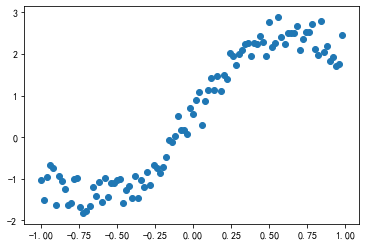然后用polyfit函数来把这些点拟合成一条3次曲线
parameter = np.polyfit(x, y, 3)

输出的结果为3次方程的参数,我们可以像下面这样把方程拼接出来
y2 = parameter * x ** 3 + parameter * x ** 2 + parameter * x + parameter

将拟合后的结果打印一下
plt.scatter(x, y)
plt.plot(x, y2, color='g')
plt.show()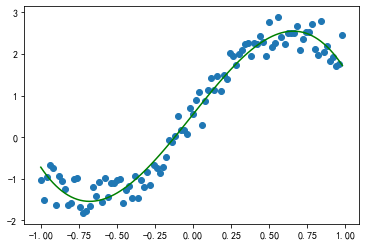还可以使用poly1d()函数帮我们拼接方程,结果是一样的
p = np.poly1d(parameter)
plt.scatter(x, y)
plt.plot(x, p(x), color='g')
plt.show()评估指标R方
二维散点进行任意函数的最小二乘拟合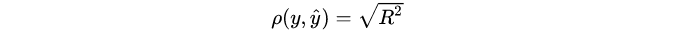最小二乘中相关系数与R方的关系推导其中，利用相关系数矩阵计算R方
correlation = np.corrcoef(y, y2)[0,1]  #相关系数
correlation**2   #R方

先来看下poly1d函数自带的输出结果
p = np.poly1d(parameter,variable='x')
print(p)

输出为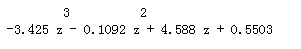这里是把结果输出到两行里了，但是输出到两行是非常不方便的
尝试下自己编写函数，使输出到一行里
parameter=[-2.44919641, -0.01856314,  4.12010434,  0.47296566]  #系数
aa=''
deg=3
for i in range(deg+1):
bb=round(parameter[i],2)     #bb是i次项系数
if bb>=0:
if i==0:
bb=str(bb)
else:
bb=' +'+str(bb)
else:
bb=' '+str(bb)
if deg==i:
aa=aa+bb
else:
aa=aa+bb+'x^'+str(deg-i)
print(aa)

输出为：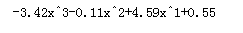封装成函数
def Curve_Fitting(x,y,deg):
parameter = np.polyfit(x, y, deg)    #拟合deg次多项式
p = np.poly1d(parameter)             #拟合deg次多项式
aa=''                               #方程拼接  ——————————————————
for i in range(deg+1):
bb=round(parameter[i],2)
if bb>0:
if i==0:
bb=str(bb)
else:
bb='+'+str(bb)
else:
bb=str(bb)
if deg==i:
aa=aa+bb
else:
aa=aa+bb+'x^'+str(deg-i)    #方程拼接  ——————————————————
plt.scatter(x, y)     #原始数据散点图
plt.plot(x, p(x), color='g')  # 画拟合曲线
# plt.text(-1,0,aa,fontdict={'size':'10','color':'b'})
plt.legend([aa,round(np.corrcoef(y, p(x))[0,1]**2,2)])   #拼接好的方程和R方放到图例
plt.show()
#    print('曲线方程为：',aa)
#    print('     r^2为：',round(np.corrcoef(y, p(x))[0,1]**2,2))

利用封装的函数重新画图
Curve_Fitting(x,y,3)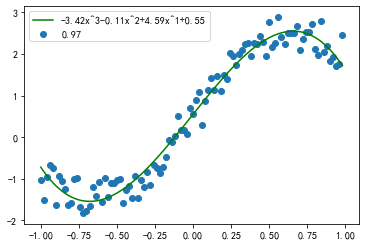展开全文python
• 神经网络入门的应用就是拟合方程，这篇文章就针对这个问题来熟悉pytorch怎么搭建神经网络模型。 1. 问题提出 我们要拟合的是y = x^2这个最简单的一元次方程，首先要创建我们的x,y数据,它们都是100*1维的数据，对y...
神经网络入门的应用就是拟合方程，这篇文章就针对这个问题来熟悉pytorch怎么搭建神经网络模型。
1. 问题提出
我们要拟合的是y = x^2这个最简单的一元二次方程，首先要创建我们的x,y数据,它们都是100*1维的数据，对y加了一个噪声，让它具有一些离散性。
# 建立数据:拟合y = x^2曲线
x = torch.unsqueeze(torch.linspace(-1, 1, 100), dim=1)  # x data (tensor), shape=(100, 1)
y = x.pow(2) + 0.2*torch.rand(x.size())                 # noisy y data (tensor), shape=(100, 1)

用散点图显示出来：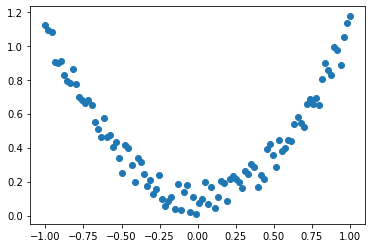2. 神经网络框架建立
pytorch中我们需要写一个类来表示神经网络的框架，它需要继承pytorch框架中的nn.Module这个类。
由于这个回归问题比较简单，我们只建立两层全连接网络结构，全连接层在nn.Module这个类中已经实现，我们只需要进行调用就好。
构造函数__init__ 是确定我们的网络架构，需要给出输入、输出层的个数和隐藏层神经单元数。
自定义的forward函数 则是我们需要自己实现的神经网络前向传播功能，在第一层隐藏层后加了激活函数relu()。
# 建立神经网络框架
class Net(nn.Module):
def __init__(self,n_feature,n_hidden,n_output):    # 构造函数
super(Net,self).__init__()                     # 继承nn.Module
# 定义每层用什么样的形式
self.hidden = nn.Linear(n_feature,n_hidden)    # 建立隐藏层
self.predict = nn.Linear(n_hidden,n_output)    # 建立输出层

# 实现前向传播功能
def forward(self,x):
x = self.hidden(x)
x = F.relu(x)    # 激活函数
x = self.predict(x)
return x

3. 神经网络训练模型的建立
在网络框架搭建完成后，要进行神经网络训练模型的建立，其主要包含3个部分：
神经网络模型建立： 就是用我们搭建的框架生成一个模型。给定各个网络层的神经单元数。
input_dim  = 1       # 输入层单元数
hidden_dim = 10      # 隐藏单元数
out_dim = 1          # 输出层单元数

net = Net(input_dim,hidden_dim,out_dim)  # 建立神经网络模型net
print(net)

可以通过打印网络模型查看其结构，输出为：
Net(
(hidden): Linear(in_features=1, out_features=10, bias=True)
(predict): Linear(in_features=10, out_features=1, bias=True)
)

优化器的建立： 选用优化器的种类，传入网络参数，设置学习率。直接用pytorch框架中封装好的优化器。
optimizer = torch.optim.SGD(net.parameters(),lr = 0.2)
# 优化器种类：SGD
# 传入参数：net.parameters()
# 学习率：lr = 0.2

损失函数的定义： 也是直接使用pytorch框架中封装好的损失函数，这里选用了均方误差MSE。
loss_func = torch.nn.MSELoss()

4. 模型的训练
由于数据简单，我们只训练100次，网络的训练模式很固定，直接看代码：
# 训练
for i in range(100):
prediction = net(x)  # 喂给net训练数据 x, 输出预测值

loss = loss_func(prediction,y)  # 计算预测值和y两者的误差
# 训练网络三个最主要步骤
loss.backward()         # 误差反向传播
optimizer.step()        # 神经网络参数更新

要注意的是每次训练的梯度要手动进行清0，因此训练代码中的最后3行是一定要有的，否则不能完成训练。
5. 结果展示
到这里，神经网络拟合方程就结束了，下面就是展示的部分了，我们可以选择输出训练过程的loss值图像。发现损失值是在不断减小的，也就意味着模型拟合的越来越好。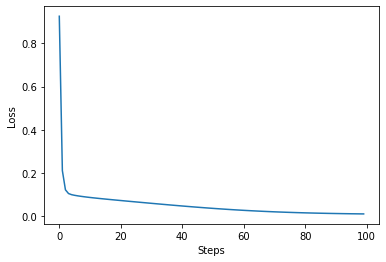让我们再看下拟合的方程，红线代表拟合过程中的方程：6. 结语
这只是最简单的神经网络应用，代码参考了莫烦大神的教程，完整代码放在这里：
# 神经网络的搭建--回归任务 #
import torch
import torch.nn.functional as F     # 激励函数都在这
import torch
import matplotlib.pyplot as plt

# 建立数据
x = torch.unsqueeze(torch.linspace(-1, 1, 100), dim=1)  # x data (tensor), shape=(100, 1)
y = x.pow(2) + 0.2*torch.rand(x.size())                 # noisy y data (tensor), shape=(100, 1)

# 建立神经网络
class Net(torch.nn.Module):  # 继承 torch 的 Module
def __init__(self, n_feature, n_hidden, n_output):
super(Net, self).__init__()     # 继承 __init__ 功能
# 定义每层用什么样的形式
self.hidden = torch.nn.Linear(n_feature, n_hidden)   # 隐藏层线性输出
self.predict = torch.nn.Linear(n_hidden, n_output)   # 输出层线性输出

def forward(self, x):   # 这同时也是 Module 中的 forward 功能
# 正向传播输入值, 神经网络分析出输出值
x = F.relu(self.hidden(x))      # 激励函数(隐藏层的线性值)
x = self.predict(x)             # 输出值
return x

net = Net(n_feature=1, n_hidden=10, n_output=1)

print(net)  # net 的结构

# 训练网络
# optimizer 是训练的工具
optimizer = torch.optim.SGD(net.parameters(), lr=0.2)  # 传入 net 的所有参数, 学习率
loss_func = torch.nn.MSELoss()      # 预测值和真实值的误差计算公式 (均方差)

plt.ion()   # 画图
plt.show()

for t in range(100):
prediction = net(x)     # 喂给 net 训练数据 x, 输出预测值

loss = loss_func(prediction, y)     # 计算两者的误差

loss.backward()         # 误差反向传播, 计算参数更新值
optimizer.step()        # 将参数更新值施加到 net 的 parameters 上

# 可视化训练过程
if t % 5 == 0:
plt.cla()
plt.scatter(x.data.numpy(), y.data.numpy())
plt.plot(x.data.numpy(), prediction.data.numpy(), 'r-', lw=5)
plt.text(0.5, 0, 'Loss=%.4f' % loss.data.numpy(), fontdict={'size': 20, 'color':  'red'})
if t<90:
plt.pause(0.1)
else:
plt.pause(1)


展开全文深度学习 python 神经网络
• 例如:自由度为2,那么拟合出来的曲线就是次函数,自由度是3,拟合出来的曲线就是3次函数 首先我们先来构造一下需要被拟合的散点 # 解决坐标轴刻度负号乱码 plt.rcParams['axes.unicode_minus'] = Fal
polyfit()函数可以使用最小二乘法将一些点拟合成一条曲线.
numpy.polyfit(x, y, deg, rcond=None, full=False, w=None, cov=False)
# x:要拟合点的横坐标
# y:要拟合点的纵坐标
# deg:自由度.例如:自由度为2,那么拟合出来的曲线就是二次函数,自由度是3,拟合出来的曲线就是3次函数

首先我们先来构造一下需要被拟合的散点
# 解决坐标轴刻度负号乱码
plt.rcParams['axes.unicode_minus'] = False
# 解决中文乱码问题
plt.rcParams['font.sans-serif'] = ['Simhei']

import numpy as np
import matplotlib.pyplot as plt
x = np.arange(-1, 1, 0.02)
y = 2 * np.sin(x * 2.3) + np.random.rand(len(x))

然后打印一下看看
plt.scatter(x, y)
plt.show()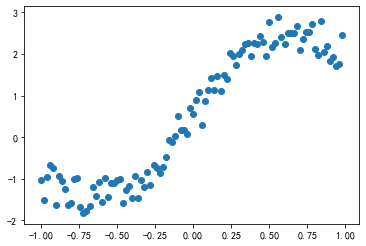然后用polyfit函数来把这些点拟合成一条3次曲线
parameter = np.polyfit(x, y, 3)

输出的结果为3次方程的参数,我们可以像下面这样把方程拼接出来
y2 = parameter * x ** 3 + parameter * x ** 2 + parameter * x + parameter

将拟合后的结果打印一下
plt.scatter(x, y)
plt.plot(x, y2, color='g')
plt.show()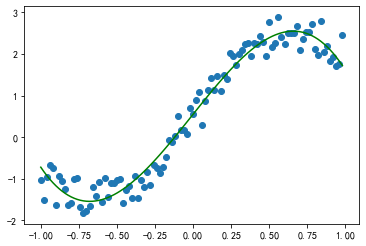还可以使用poly1d()函数帮我们拼接方程,结果是一样的
p = np.poly1d(parameter)
plt.scatter(x, y)
plt.plot(x, p(x), color='g')
plt.show()评估指标R方
二维散点进行任意函数的最小二乘拟合最小二乘中相关系数与R方的关系推导其中，利用相关系数矩阵计算R方
correlation = np.corrcoef(y, y2)[0,1]  #相关系数
correlation**2   #R方

先来看下poly1d函数自带的输出结果
p = np.poly1d(parameter,variable='x')
print(p)这里是把结果输出到两行里了，但是输出到两行是非常不方便的
尝试下自己编写函数，使输出到一行里
parameter=[-2.44919641, -0.01856314,  4.12010434,  0.47296566]  #系数
aa=''
deg=3
for i in range(deg+1):
bb=round(parameter[i],2)     #bb是i次项系数
if bb>=0:
if i==0:
bb=str(bb)
else:
bb=' +'+str(bb)
else:
bb=' '+str(bb)
if deg==i:
aa=aa+bb
else:
aa=aa+bb+'x^'+str(deg-i)
print(aa)封装成函数
def Curve_Fitting(x,y,deg):
parameter = np.polyfit(x, y, deg)    #拟合deg次多项式
p = np.poly1d(parameter)             #拟合deg次多项式
aa=''                               #方程拼接  ——————————————————
for i in range(deg+1):
bb=round(parameter[i],2)
if bb>0:
if i==0:
bb=str(bb)
else:
bb='+'+str(bb)
else:
bb=str(bb)
if deg==i:
aa=aa+bb
else:
aa=aa+bb+'x^'+str(deg-i)    #方程拼接  ——————————————————
plt.scatter(x, y)     #原始数据散点图
plt.plot(x, p(x), color='g')  # 画拟合曲线
# plt.text(-1,0,aa,fontdict={'size':'10','color':'b'})
plt.legend([aa,round(np.corrcoef(y, p(x))[0,1]**2,2)])   #拼接好的方程和R方放到图例
plt.show()
#    print('曲线方程为：',aa)
#    print('     r^2为：',round(np.corrcoef(y, p(x))[0,1]**2,2))

利用封装的函数重新画图
Curve_Fitting(x,y,3)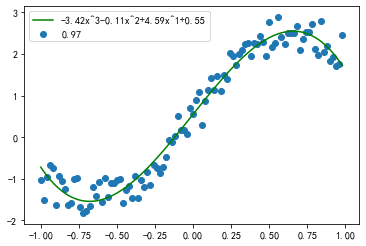展开全文python
• C语言实现拟合直线方程在进行拟合直线的时候，最一般的做法是最小二乘法，此方法比较快速，易懂，下列程序用最小二乘法拟合直线。
在进行拟合直线的时候，最一般的做法是最小二乘法，此方法比较快速，易懂。
理论：对于点集

{(xi,yi)|i=1,2,3…n}
$\{(x_i, y_i) | i=1,2,3 \dots n\}$，设其直线方程为

ax+by+c=0
$ax+by+c=0$。一般来讲，直线可由两个未知数来表示，设

b=1
$b=1$，则直线的方程为：

ax+y+c=0
$ax+y+c=0$。最小二乘法力图使优化目标函数

f=∑i=1n(axi+byi+c)2

f = \sum_{i=1}^n (ax_i+by_i+c)^2 的值最小。

上式分别对a和c求偏导，有：

⎧⎩⎨⎪⎪⎪⎪∂f∂a=2∑(axi+yi+c)xi=0∂f∂c=2∑(axi+yi+c)=0

\left\{\begin{array}{c}
\frac{\partial f}{\partial a}=2\sum(ax_i+y_i+c)x_i=0 \\
\frac{\partial f}{\partial c}=2\sum(ax_i+y_i+c) = 0
\end{array}\right.
则有：

⎛⎝∑x2i∑xi∑xi∑1⎞⎠A(ac)C=⎛⎝∑xiyi∑yi⎞⎠B

\underbrace{\begin{pmatrix}\sum x_i^2 & \sum x_i\\\sum x_i & \sum 1\end{pmatrix}}_A\underbrace{\begin{pmatrix}a\\c\end{pmatrix}}_C = \underbrace{\begin{pmatrix}\sum x_iy_i\\\sum y_i\end{pmatrix}}_B

程序：
void myFFitLine1D(const double *nDataX, const double *nDataY, const int nLength, double &a, double &b,double &c)
{
//直线的方程为：AX+BY+C=0,一般来讲，A^2+B^2 != 0,而A,B,C均有可能为0，
//以下程序利用最小二乘原理，将上述中
//直线方程分为：AX+Y+C=0和X+BY+C=0两种，并在最后作规一化，即A^2+B^2=1
//1. 当B为1时，直线与点的方程可写为：AX+C=-Y,即有：
// |x1,x2,x3| |x1,1| |A| = -|x1,x2,x3| |y1|
// |1 , 1, 1| |x2,1| |C|    |1 , 1, 1| |y2|
//            |x3,1|                   |y3|
//     {A}           {C}         {B}
//2. 当A为1时，同样可以得到类似的结论

double A = {0};
double B = {0};
double C = {0};

if(abs(nDataX[nLength-1] - nDataX) > abs(nDataY[nLength-1] - nDataY))
{
for(int i=0;i<nLength;i++)
{
A += nDataX[i]*nDataX[i];
A += nDataX[i];

B -= nDataX[i] * nDataY[i];
B -= nDataY[i];
}

}
else
{
for(int i=0;i<nLength;i++)
{
A += (*(nDataY+i))*(*(nDataY+i));
A += (*(nDataY+i));

B -= (*(nDataX+i)) * (*(nDataY+i));
B -= (*(nDataX+i));
}
}
A = A;
A = nLength;

double DetA = A*A - A*A;
if(abs(DetA) < 0.0000001)
return;
C =  (A*B - A*B) / DetA;
C = (-A*B + A*B) / DetA;

if(abs(nDataX[nLength-1] - nDataX) > abs(nDataY[nLength-1] - nDataY))
{
a = C;   b = 1;  c = C;
}
else
{
a = 1;  b = C;   c = C;
}
//对直线方程进行规一化，即A^2+B^2 = 1. 由于a或b为1，此处不进行除0检查
DetA = a*a + b*b;
a = a / DetA;
b = b / DetA;
c = c / DetA;
}

展开全文• plt.show() 还可以使用poly1d()函数帮我们拼接方程,结果是一样的 p = np.poly1d(parameter) plt.scatter(x, y) plt.plot(x, p(x), color='g') plt.show() 评估指标R方 维散点进行任意函数的最小二乘拟合 ...
• 多项式拟合之一般方程法 文章目录多项式拟合之一般方程法1 什么是多项式拟合？2 怎样拟合？3 求bbb正交矩阵QR分解施密特正交化matlab测试函数 之前经常会用到多项式拟合拟合函数关系，十分好用，得出的表达式使用...matlab 多项式 机器学习
• ## 二维曲线拟合

千次阅读 2018-11-20 16:49:03
维曲线拟合相关基础理论知识最小二乘法广义逆（伪逆矩阵）矩阵分解特征值分解（Eigen Value Decomposition，EVD）Householder变换法（QR Factorization,QR分解）奇异值分解（singular value decomposition,SVD）...
• 第二章Probability Distributions的贝塔-二项式、狄利克雷-多项式共轭、高斯分布、指数族等很基础也很重要。伯努利分布 高斯分布
• 常见的曲线拟合方法   1.使偏差绝对值之和最小 ...按偏差平方和最小的原则选取拟合曲线，并且采取二项式方程拟合曲线的方法,称为最小二乘法。 多项式拟合介绍 多项式拟合公式 多项式拟合问题描述 假定机器学习
• 维/三维直线拟合–opencv实现 ​ Hough变换可以提取图像中的直线，但是提取线的精度不高，然而我们的很多场合下需要精确估计直线的参数，这时就需要进行直线拟合。 ​ 直线拟合的方法有很多，比如：一元线性回归...
• 在上一篇的博客球面数据拟合算法简介中，笔者详细介绍了关于空间球面数据拟合的算法公式推导并给出了相应的Matlab代码及其仿真分析。本次笔者将上面这一情况进行更一般的推广，即取消了球面数据这一限制，数据可以是...数据 算法 matlab
•   多项式拟合（polynominal curve fitting）是一种线性模型，模型和拟合参数的关系是线性的。多项式拟合的输入是一维的，即x=xx=x\textbf{x} = x，这是多项式拟合和线性回归问题的主要区别之一。   多项式拟合...线性 最小二乘
• % 误差求和方程，这里是提供的值对应的函数值。 % 分别对不同系数求导数： % f(x) = a2*x^2 + a1*x + a0 A = []; % 最小二乘法求拟合曲线系数 for k=1:1:3 for g=1:1:4 sumdata1 = 0; for j=1:1:11 ...
• ENVI/IDL （5.3版本）一键化实现LST-NDVI的干湿边方程拟合，并得到TVDI计算结果图 0 原理介绍 利用IDL将NDVI异常值进行剔除，NDVI取值范围为0.2~1(植被覆盖区)，对反演的地表温度Ts选择5%的置信区间，构建NDVI-Ts...
• ## 数据拟合

万次阅读 2014-11-04 09:56:14
假设对观测数据进行拟合，得到的拟合曲线为。将观测数据代入，得到，其和的偏差定义为   （1） 评价拟合结果好坏的函数称为指标函数
• 图三是过拟合 特征选择的方式有过滤（低方差特征），嵌入式（正则化，决策树，神经网络） 正则化的使用：尽量减少高次的权重。 L2正则化： 在sklearn的linear_model中，有一个Ridge，是代表带有正则化的线性回归...
• 本文主要以左右车道检测方法中基于车道侧面连续的曲线拟合方法进行研究。 通常的车道检测方法分两步：（1）特征提取（2）车道几何模型的建立与匹配 在车道检测问题探究（一）车道特征提取（上） ...算法 algorithm filter 优化
• 多项式拟合C# Class1.cs-拟合类 using System; using System.Collections.Generic; using System.Text; namespace 最小二乘法拟合多项式 { class Class1 { ///&lt;summary&gt; ///用最小二乘法拟合二元...多项式
• ## C++——多项式拟合

千次阅读 多人点赞 2018-07-20 15:37:32
C++——多项式拟合 目标：利用C++对txt或者xml中的数据，进行高阶或低阶多项式拟合  为方便以后查找，代码以及详细资料已打包，并上传至云盘（链接：https://pan.baidu.com/s/1bvUBIoxv7Avxeq_Cz6xOZQ 密码：u9qe...
• 机器学习之过拟合，欠...过拟合发生的本质原因，是由于监督学习问题的不适定：在高中数学我们知道，从n个（线性无关）方程可以解n个变量，解n+1个变量就会解不出。在监督学习中，往往数据（对应了方程）远远少于模...l1
• 怀着沉痛的心情，拖着疲惫的身心，为了拟合好圆，我实在不得不上最小二乘法了(我上班写的代码不要想了，不可能发在blog里的)，现在进入正题。 （1）基本原理 名称 自变量：x ...opencv
• ## 曲线拟合

万次阅读 2016-08-29 10:26:14
12.1 曲线拟合 ...曲线拟合通俗的说法就是“拉曲线”，也就是将现有数据透过数学方法来代入一条数学方程式的表示方法。科学和工程遇到的很多问题，往往只能通过诸如采样、实验等方法获得若干离
• #ecoding=utf-8 import matplotlib.pyplot as plt #生成离散x x = np.arange(-10 , 10 , 2) ...#二项式拟合 pfit = np.polyfit(x , y , 2) y_fun = np.poly1d(pfit) plt.plot(x, y,'*', color='r...
• 五、插值与拟合插值和拟合都是要根据一组数据构造一个函数作为近似，但插值和拟合的近似要求不同，注意区分。插值：在平面上给定一组离散点列满足函数 ，要求一条近似曲线 来替代目标函数，且近似曲线需经过所有已知...
• 如下图所示，第一行是X，第一列是Y，其余为Z，欲拟合Z（X，Y）的次关系。 matlab代码 注意，要将X 和Y 扩充至与Z同维！！！！ %matlab clc;clear; x=[0. 1000. 1285.714286 1571.428571 1857.142857 2142....
• 目录 拟合与岭回归 ...1 什么是过拟合与欠拟合 2 模型复杂度 3 鉴别欠拟合与过拟合 4 过拟合解决方法 5 岭回归（Ridge） 6 模型的保存与加载 拟合与岭回归 1 什么是过拟合与欠拟合 通过下面两...线性回归
• MATLAB软件提供了基本的曲线拟合函数的命令． 多项式函数拟合：a=polyfit(xdata,ydata,n) 其中n表示多项式的最高阶数，xdata，ydata为将要拟合的数据，它是用数组的方式输入．输出参数a为拟合多项式 的系数 ...matlab...